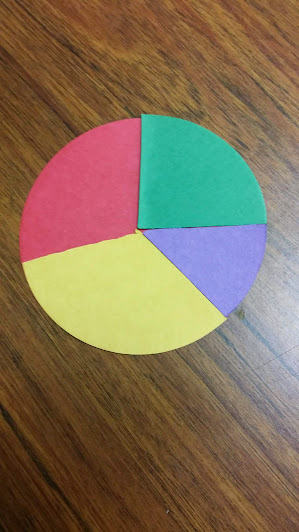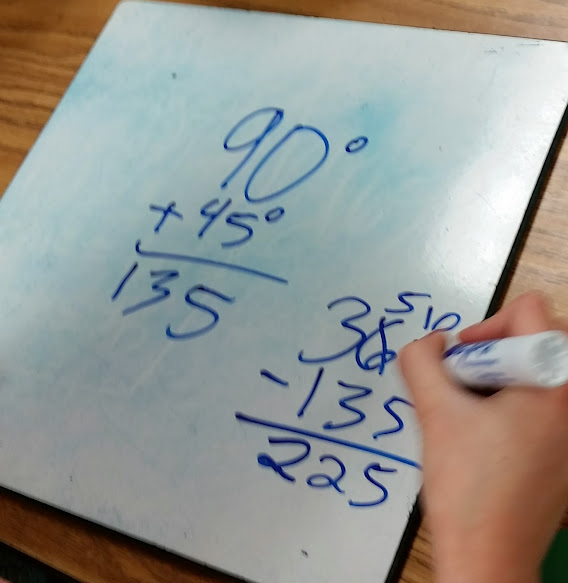# Rainbow of Angles!

4 teachers like this lesson
Print Lesson

## Objective

SWBAT explore angles and their measurements using 360 degree circles and parts of circles to make sense of benchmark angles.

#### Big Idea

In this lesson, students use fraction circles and an interactive smart board program to explore angle measurements as part of 360 degrees of circles.

## Warm Up

7 minutes

To begin this lesson, I review important geometry terms with students like - angle, acute angle, obtuse angle, right angle, ray, line, point.  I review these terms by listing a word and asking students to give a thumbs up if they know the word and can explain it to their partner. This is a great way to informally check for understanding.  Then, I ask one student volunteer to share their definition.

This is important when thinking about scaffolding and gradual release of my students. At this point, I do not assume that my students know these terms, but I know they are familiar with them. I can also help clear up any misunderstanding or confusion about the terms as students tell me what they know and remember about each term.

## Concept Development

45 minutes

I start this lesson with a discussion about what students already know about angles, especially those that are more familiar to them, such as right angles. Then I give each student one set of fraction circles - One red whole circle, an orange half circle, three yellow 120 degree wedges or 1/3 pieces, four green 90 degree wedges or four 1/4 pieces, six blue 60 degree angles or six 1/6 pieces, and eight purple 45 degree pieces or eight 1/8 pieces.

One way students can connect to this idea is by talking about skateboard and snowboard tricks, like the 180 and the 360, and what kind of movements are made in those tricks.

Next, I ask students, “If you divide a circle in half how many degrees would that represent?” (180°). I ask students if they  could jump all the way around in one jump would they be doing a 360?  Students respond with, Yes!

Next, I tell students that it makes sense that there are 360 degrees in a circle. I explain that they will be exploring the degrees in a circle and how that relates to angle measures. I explain that when we talk about angles (as opposed to temperature), a degree is a unit used to measure an angle between two line segments or rays.  Degrees can also measure rotation, how far something has been rotated from  a starting point.

Then I tell my students some of the history of angles. The idea of measuring angles in degrees was invented about 3700 years ago in ancient Babylonia. The Babylonians worked in a base sixty system. (One of the reasons we work in base ten is because we have 10 fingers.) No one is quite sure why the Babylonians worked in base sixty. Can you think of any good reasons to use that number? The Babylonians divided the circle into 360 parts, calling each tiny part a degree. How does 360 relate to 60? (6 × 60 is 360) Half of 360 is 180, so it takes 180 degrees to rotate halfway around a circle. Along with degree measure, we have inherited from the Babylonians two other measures that use 60. Can you think of what they are? (60 minutes in an hour and 60 seconds in a minute)

Next, I instruct students to take out their whole circle (red).   I ask students how much of the circle would be covered up if we rotated 180 degrees. Students respond with half and I ask them to show that with the orange fraction piece.

Next, on the board, I write 360 ÷ 2 = 180.

Then I ask students how much of the circle would be covered up if we rotated 120 degrees. Students respond with one third of the circle, and also show that with the yellow fraction pieces. I also write on the board 360 ÷ 3 = 120.

I continue, like above, with all other wedges, asking students how much of the circle would be covered up each time.  Students respond with 90 degrees, 60 degrees, and 45 degrees. Students use Math Practice 7 - look for and make use of structure when they begin to see the relationships between the number of degrees in a whole circle divided by the total number of parts in order to determine the angle measurement of each wedge.After students have discovered the angle measurements of each wedge, I then put different colored wedges on the red circle and ask students to figure out the angle created.  You can see in the photo below a student determining the angle created by a green wedge and purple wedge, and then determining the supplementary angle created.In this video, you can also hear a student talking about how she is determining the angle measurements of various wedges.

For the last part of this lesson, I use an interactive whiteboard protractor located here.  I specifically chose to have students spend the entire class period with manipulatives to build conceptual understanding about measuring angles. So often, students are taught a procedure, (how to measure angles) with little understanding about why the procedure works. I want my students to understand the relationship between circles and angles.

Using just the angle part of this tool, (no protractors yet) I display various angles and ask students to estimate the degree of the angle based on the knowledge they learned today.  If students seem to struggle, this tool allows me to show a circle as well as the angle. This really helps students solidify the idea that an angle's rays intersect at point, and that point is also the mid point of a circle, which is what degrees are based on.